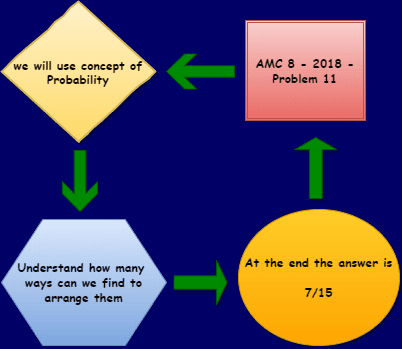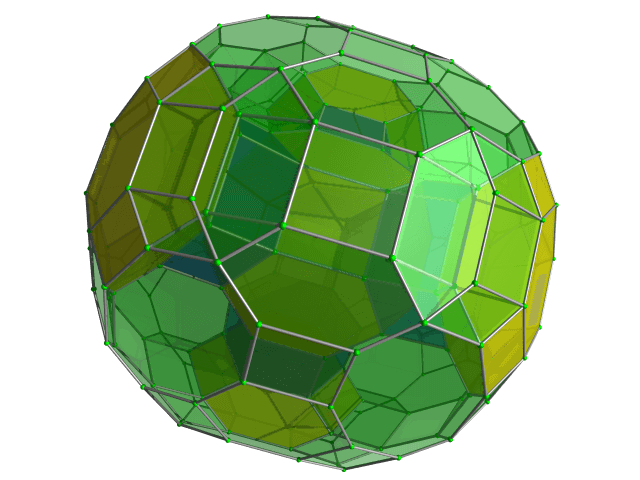Categories

# Probability- AMC 8, 2018 – Question 11

Try this beautiful problem from AMC 8. It involves basic statistics and data representation. We provide sequential hints so that you can try the problem.

## Competency in Focus: Concept of Probability

This problem from American Mathematics Contest 8 (AMC 8, 2018) is based on calculation of probability. It is Question number 11 of the AMC 8 2018 Problem series.

## First look at the knowledge graph:-## Next understand the problemAbby, Bridget, and four of their classmates will be seated in two rows of three for a group picture, as shown. If the seating positions are assigned randomly, what is the probability that Abby and Bridget are adjacent to each other in the same row or the same column? $\textbf{(A) } \frac{1}{3} \qquad \textbf{(B) } \frac{2}{5} \qquad \textbf{(C) } \frac{7}{15} \qquad \textbf{(D) } \frac{1}{2} \qquad \textbf{(E) } \frac{2}{3}$

### Basic Probability sum

6/10
##### Suggested Book
Challenges and Thrills in Pre College Mathematics Excursion Of Mathematics

Do you really need a hint? Try it first!
If you need any hint try from this: There are a total of $6!$ ways to arrange the kids.
Abby and Bridget can sit in 3 ways if they are adjacent in the same column:For each of these seat positions, Abby and Bridget can switch seats, and the other 4 people can be arranged in $4!$ ways which results in a total of $3 \times 2 \times 4!$ ways to arrange them.
By the same logic, there are 4 ways for Abby and Bridget to placed if they are adjacent in the same row, they can swap seats, and the other $4$ people can be arranged in $4!$ ways, for a total of $4 \times 2 \times 4!$ ways to arrange them.
We sum the 2 possibilities up to get $\frac{(3\cdot2)\cdot4!+(4\cdot2)\cdot4!}{6!} = \frac{14\cdot4!}{6!}=\boxed{\frac{7}{15}}$

## AMC - AIME Program

AMC - AIME - USAMO Boot Camp for brilliant students. Use our exclusive one-on-one plus group class system to prepare for Math Olympiad## External Tangent | AMC 10A, 2018 | Problem 15

Try this beautiful Problem on geometry based on circle from AMC 10A, 2018. Problem-15. You may use sequential hints to solve the problem.

## Dice Problem | AMC 10A, 2014| Problem No 17

Try this beautiful Problem on Probability from AMC 10A, 2014. Problem-17, You may use sequential hints to solve the problem.

## Problem on Curve | AMC 10A, 2018 | Problem 21

Try this beautiful Problem on Co-ordinate geometry from AMC 10A, 2018. Problem-21, You may use sequential hints to solve the problem.

## Right-angled Triangle | AMC 10A, 2018 | Problem No 16

Try this beautiful Problem on triangle from AMC 10A, 2018. Problem-16. You may use sequential hints to solve the problem.

## Finding Greatest Integer | AMC 10A, 2018 | Problem No 14

Try this beautiful Problem on Algebra from AMC 10A, 2018. Problem-14, You may use sequential hints to solve the problem.

## Length of the crease | AMC 10A, 2018 | Problem No 13

Try this beautiful Problem on triangle from AMC 10A, 2018. Problem-13. You may use sequential hints to solve the problem.

## Right-angled shaped field | AMC 10A, 2018 | Problem No 23

Try this beautiful Problem on triangle from AMC 10A, 2018. Problem-23. You may use sequential hints to solve the problem.

## Area of region | AMC 10B, 2016| Problem No 21

Try this beautiful Problem on Geometry on Circle from AMC 10B, 2016. Problem-20. You may use sequential hints to solve the problem.

## Coin Toss Problem | AMC 10A, 2017| Problem No 18

Try this beautiful Problem on Probability from AMC 10A, 2017. Problem-18, You may use sequential hints to solve the problem.

## GCF & Rectangle | AMC 10A, 2016| Problem No 19

Try this beautiful Problem on Geometry on Rectangle from AMC 10A, 2010. Problem-19. You may use sequential hints to solve the problem.

This site uses Akismet to reduce spam. Learn how your comment data is processed.